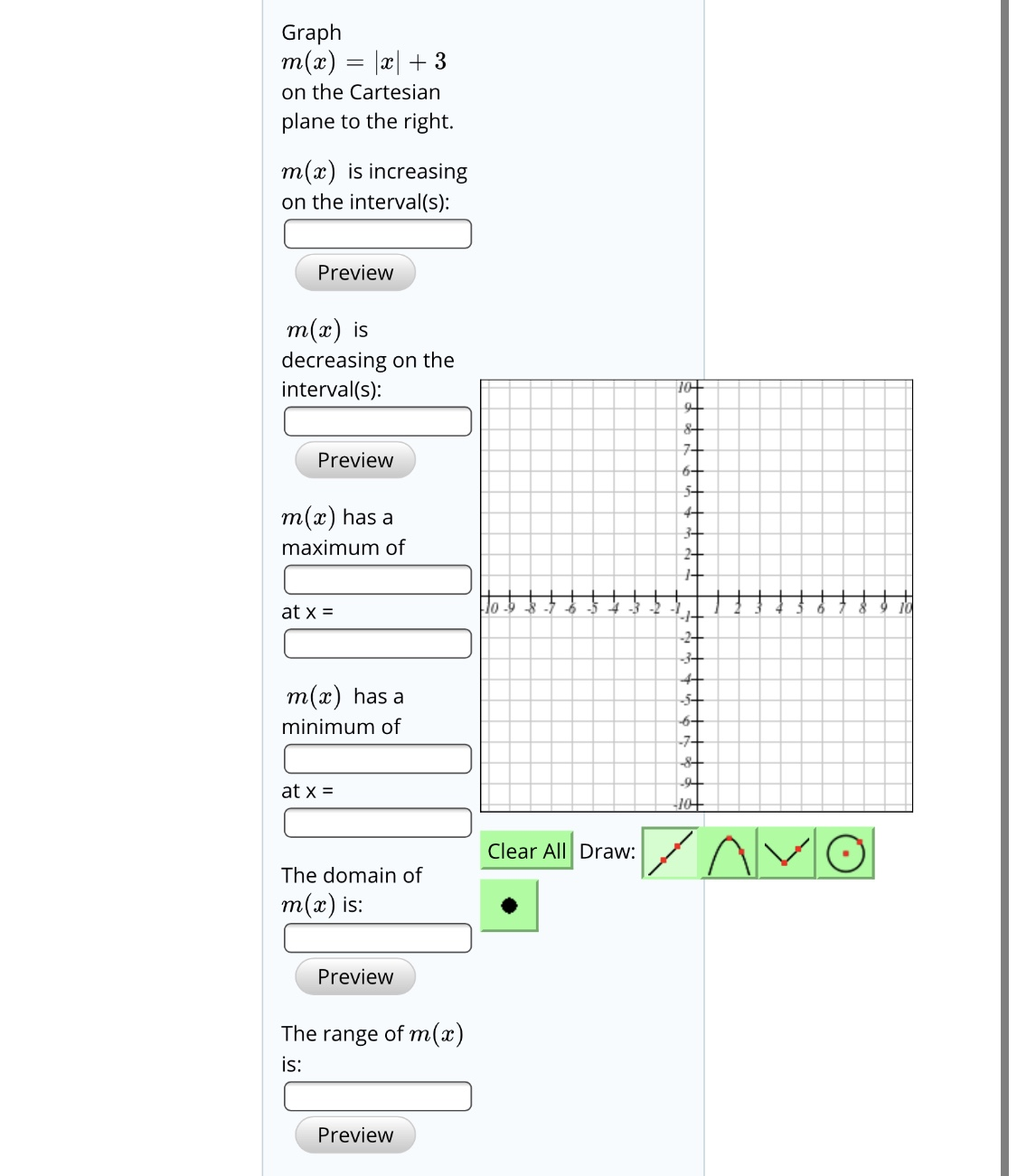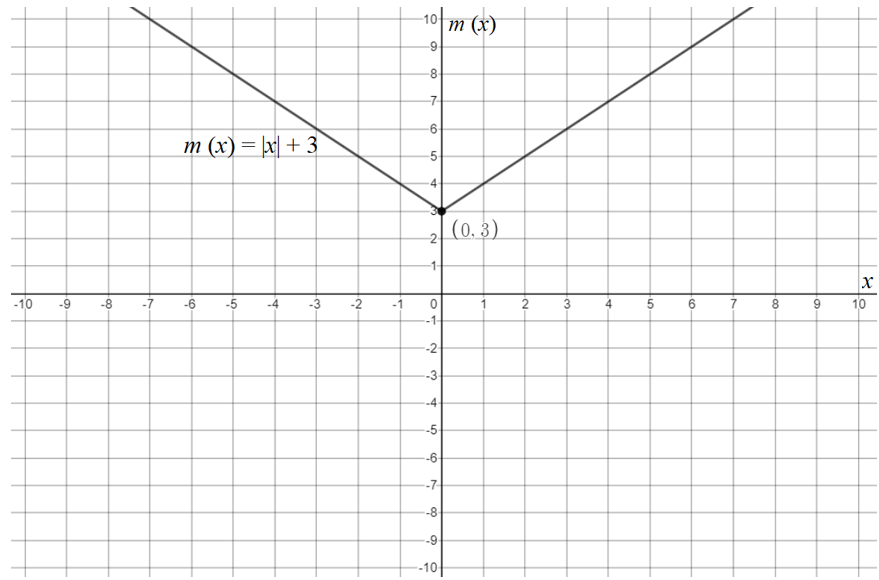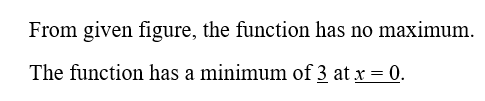# Graphm(x) = |x| + 3on the Cartesianplane to the right.m(x) is increasingon the interval(s):Previewm(x) isdecreasing on theinterval(s):107+Preview6+5+m(x) has amaximum of1010at x =-3 -2-2+3+m(x) has a-5-6+minimum of-7-at x =Clear All Draw:The domain ofm(x) is:PreviewThe range of m(x)is:Preview

Question
1 viewshelp_outlineImage TranscriptioncloseGraph m(x) = |x| + 3 on the Cartesian plane to the right. m(x) is increasing on the interval(s): Preview m(x) is decreasing on the interval(s): 10 7+ Preview 6+ 5+ m(x) has a maximum of 10 10 at x = -3 -2 -2+ 3+ m(x) has a -5- 6+ minimum of -7- at x = Clear All Draw: The domain of m(x) is: Preview The range of m(x) is: Preview fullscreen
check_circle

The graph of the given function is shown in below figure.Step 2...

### Want to see the full answer?

See Solution

#### Want to see this answer and more?

Solutions are written by subject experts who are available 24/7. Questions are typically answered within 1 hour.*

See Solution
*Response times may vary by subject and question.
Tagged in